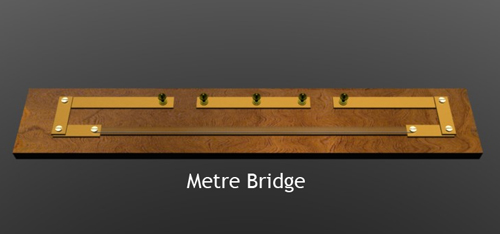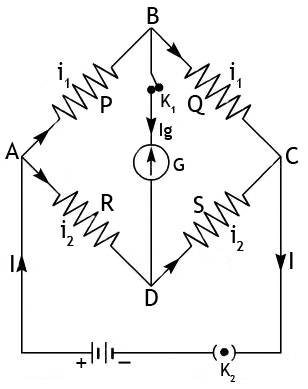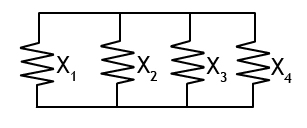Metre bridge- Law of combination of resistors

# Our Objective:

To verify the laws of resistances in series and parallel.

# The Theory:

## Metre Bridge

The metre bridge, consists of a one metre long wire of uniform cross sectional area, fixed on a wooden block. A scale is attached to the block. Two gaps are formed on it by using thick metal strips in order to make the Wheat stone’s bridge. The terminal B between the gaps is used to connect galvanometer and jockey.The metre bridge is operates under Wheatstone’s principle. Here, four resistors P, Q, R, and S are connected to form the network ABCD.

In the balancing condition, there is no deflection on the galvanometer. Then,

A resistance wire is introduced in gap G1 and the resistance box is in gap G2. One end of the galvanometer is connected to terminal D and its other end is connected to a jockey. As the jockey slides over the wire AC, it shows zero deflection at the balancing point (null point).If the length AB is l, then the length BC is ( 100-l ).

Then, according to Wheatstone’s principle;

Now, the unknown resistance can be calculated as,

## Resistors in Series

When two or more resistors are connected such a way that one end of one resistor is connected to the starting end of the other, then the circuit is called a Series Circuit.When the two resistors X1 and X2 are connected in series in a circuit, the current I passing through each resistor is same.Using Ohm’s Law, the potential difference V1 across X1 is:

Let Xs be the effective resistance of the two resistors in series, and V be the potential difference across the ends.

Thus, when a number of resistors are connected in series, the effective resistance is equal to the sum of the individual resistances. This is called the law of combination of resistances in series.

Adding resistors in series always increases the effective resistance.

## Resistors in Parallel

If the starting ends of two resistors are joined to a point and the terminal ends of the two are combined and given connection to a source of electricity,those circuits are called Parallel Circuit.When the two resistors X1 and X2 are connected in parallel in a circuit, the potential difference across X1 and X2 are the same.Then the current passing through the circuit is,

If there are ‘n’ number of resistors with different resistances connected in parallel, then we have,

That is, for a set of parallel resistors, the reciprocal of their equivalent resistance equals the sum of the reciprocals of their individual resistances. Thus, resistance decreases in parallel combination.

# Learning Outcomes:

• The student learns the following concepts:
• Resistance in a circuit.
• When two resistors are connected in series, its equivalent resistance increases.
• Law of combination of resistors connected in series.
• When two resistors are connected in parallel, its equivalent resistance decreases.
• Law of combination of resistors connected in parallel.